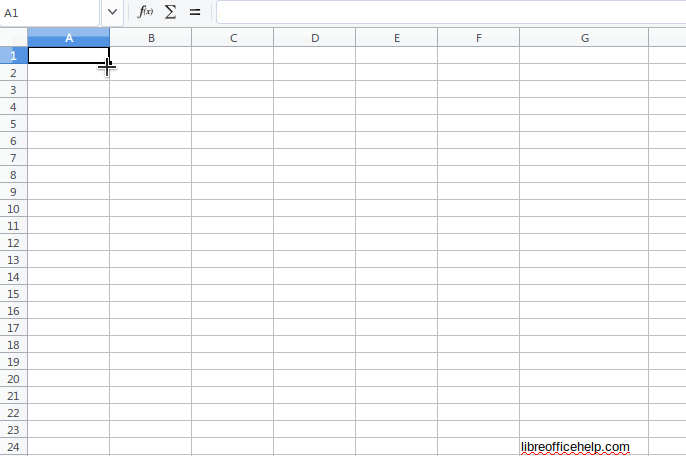Repeat a given alphanumeric sequence so many times eg abcd abcd abcd in excel

How Can I Repeat a given alphanumeric sequence so many times eg abcd abcd abcd in the spreadsheet of libre office CALC? For example: I may have in cells A1:A4 the alphanumeric string: A,B,C,D

I want to repeat that sequence in cells e.g. B1:B40 so many times. How is this possible?

A1: A    B1: A
A2: B    B2: B
A3: C    B3: C
A4: D    B4: D
B5: A
B6: B
B7: C
B8: D
B9: A
B10: B
B11: C
B12: D
B..: ...
B37: A
B38: B
B39: C
B40: D

Yes, it is possible. Have a look.Thank you for your answer. It was really very helpful. However, if someone wants to not have an extra long, numerical column, is it possible to create the same alphanumeric sequence without the appearance of that long numerical column? Have a nice day sir!

If you don’t want an extra numerical collumn I would propose the following:

B1: <sequence value 1>
B2: <sequence value 2>
B3: <sequence value 3>
B4: =B1 -> then copy cell A4 to all the cells below and voila!

Thank you Impocta!